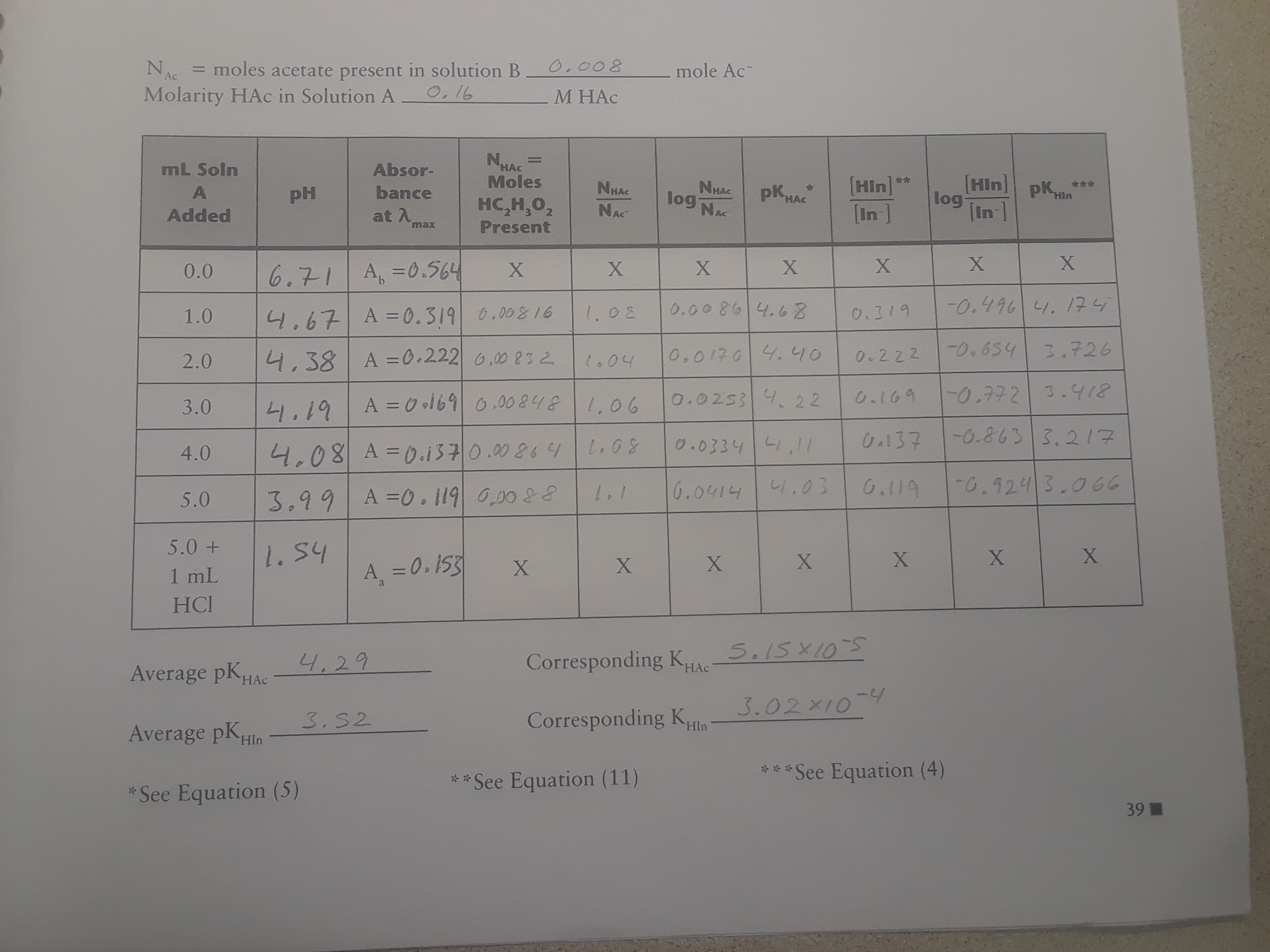# 0.008 moles acetate present in solution B O,16 mole Ac Ac Molarity HAc in Solution A М НАС N Moles mL Soln Absor- HAC [Hin In ] [Hin] NHAC log pK in ] NHAC pK A bance PH log Hin HAC HC Н,о, Present NAC NAC Added at A max X X X A,=0.564 0.0 6.71 -0.4964.17 0.00 8614.68 .02 0.00&16 0.319 1.0 4.67 A =0.319 0.654 3.726 0.01764. 1O A =0.222 6 ,00 832 4,38 0.222 2.0 l.04 0.772 3.Y18 C.169 0.0253 22 A 0 ol69 000 212,06 3.0 4.19 -0.863 3.217 0.137 0.0334 1I7 L.08 4.08 A-0.1370.0026 4.0 0.9243.066 0.119 6.0414 14403 A =0. 119 000 88 3.99 5.0 5.0+ 2.54 X X A =0.153 X 1 mL НCI 4.29 Corresponding KAe.5X10 HAc Average pKHAc 3.02x10-9 Corresponding K 3.S2 HIn Average pK HIn ***See Equation (4) **See Equation (11) See Equation (5) 39

Question

It was assumed:

a) Before any acetic acid was added, essentially all the indicator was in the In- form.

b) After the HCl was added, essentially all the indicator was in the HIn form.

Justify these assumptions using the measured pH of these solutions and your experimental value for pKHIn to determine:

1) The fraction fo the indicator in the HIn form in solution B before any of solution A was added

2) The fraction of the indicator in the In- form after the HCl was added.help_outlineImage Transcriptionclose0.008 moles acetate present in solution B O,16 mole Ac Ac Molarity HAc in Solution A М НАС N Moles mL Soln Absor- HAC [Hin In ] [Hin] NHAC log pK in ] NHAC pK A bance PH log Hin HAC HC Н,о, Present NAC NAC Added at A max X X X A,=0.564 0.0 6.71 -0.4964.17 0.00 8614.68 .02 0.00&16 0.319 1.0 4.67 A =0.319 0.654 3.726 0.01764. 1O A =0.222 6 ,00 832 4,38 0.222 2.0 l.04 0.772 3.Y18 C.169 0.0253 22 A 0 ol69 000 212,06 3.0 4.19 -0.863 3.217 0.137 0.0334 1I7 L.08 4.08 A-0.1370.0026 4.0 0.9243.066 0.119 6.0414 14403 A =0. 119 000 88 3.99 5.0 5.0+ 2.54 X X A =0.153 X 1 mL НCI 4.29 Corresponding KAe.5X10 HAc Average pKHAc 3.02x10-9 Corresponding K 3.S2 HIn Average pK HIn ***See Equation (4) **See Equation (11) See Equation (5) 39 fullscreen